# XES - Programmers Guide - parsing

The parser for this program was generated by javacc and jjtree as follows:

I have loaded Java1.5.zip from here.

• Load javacc-3.2.zip (or later version) from here. This contains both javacc and jjtree.
• run jjtree
• run javacc

This parser does not generate XES directly but generates a tree structure which this program then converts into XES.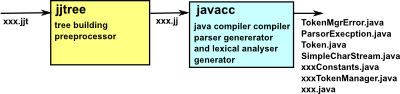I have draw out part of the tree structure this javacc parsor will create here.

The convertion between these tree structures is described below:

## Variables, declaration and assign.

Variables may be defined, initialised and used in the same statement or in seperate statements. Any variables may also be used in arrays.

All of these things are based on variable node, even assignment will allways have a varible on the left hand side.

When a variable is used we need a quick way to link it back to its definition, how do we define such a link? we need to do this both for local variables, class varibles and varibles in other classes, how do we implement late binding of variables?

Should all node names be expanded out to their full path name?

 Java example java1.5.zip tree structure XES tree structure XES ```int var1; ```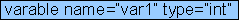`` `var1 = 1;`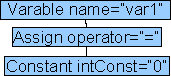``` ``` `int var2 =2;`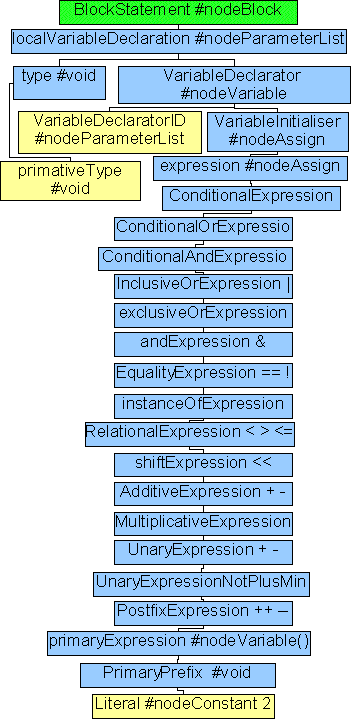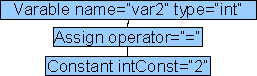``` ``` `int[] array1;`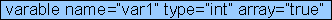`` `array1 = new int;`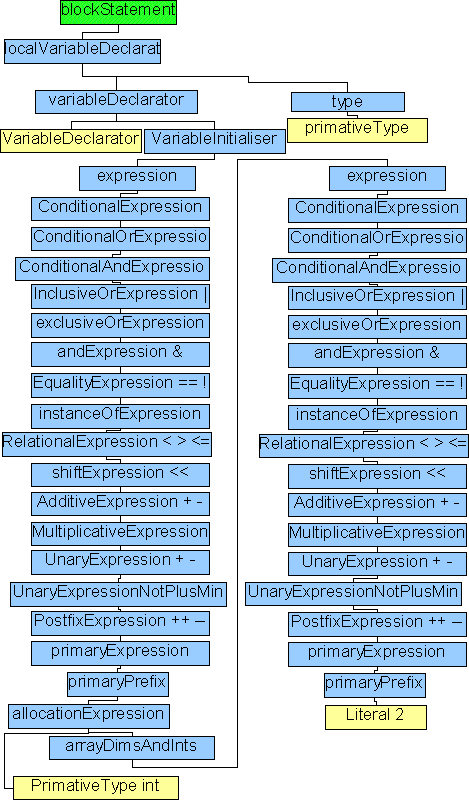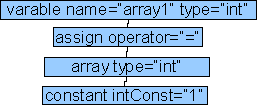``` ``` `array1=3;`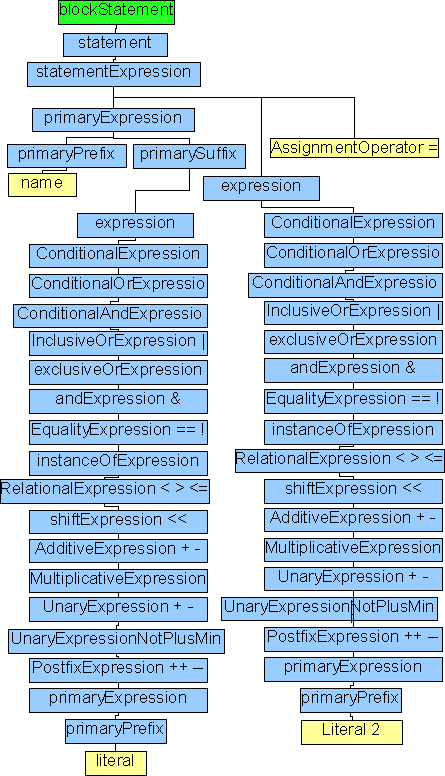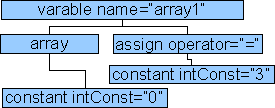``` ``` `int[] array2=new int;```` ``` double x,y,z;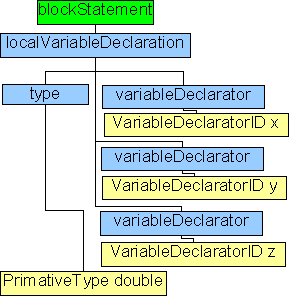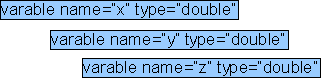`is:` `` `should be:` ```x y z``` JDK 1.5 allows Generic Types: ArrayList list = new ArrayList(); JDK 1.5 also allows enum: public enum StopLight { red, amber, green }; ``` ArrayList ``` ``` StopLight ``` String values[] = (String []) names; (String []) names other possible parameters: type = "=" default type = "++pre" type = "++post" type = "--pre" type = "--post" type = "+=" type = "-=" type = "*=" type = "/=" type = "%=" type = "&=" type = "|=" type = "^=" type = "<<=" type = ">>=" type = "<<<="

Statements

 Java example java1.5.zip tree structure XES tree structure XES `Math.abs(1);`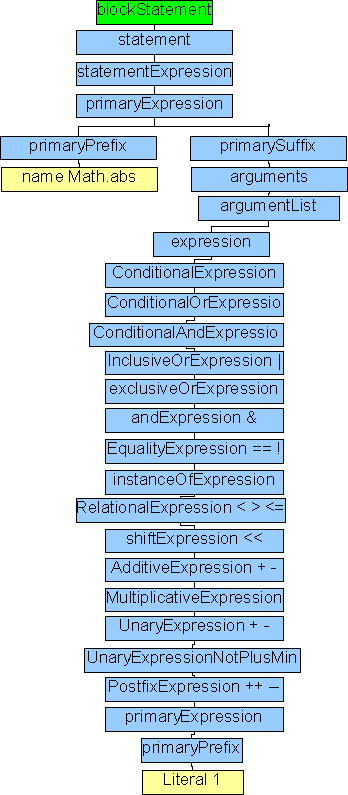` ` `a++`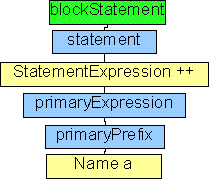` ` `++a` ` ` ` ` ` ` ` ` ` ` ` ` ` ` ` ` ` ` ` ` ` `